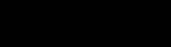# A More Versatile Loop : the while Function with autolisp in AutoCAD

The (repeat) function is limited to situations where the programmer (or the user) can determine beforehand the number of loops desired. The more common looping situation is to continue to execute a series of expressions until a certain changing condition is met. This is exactly the use of the (while) function.

Explanation: the (while) function

(while expression1
expression2…
expressionn
)

where expressionx is a conditional statement.

The (while) function first evaluates the expression1. As long as it is non- nil, the remaining expressions are evaluated and it then re-evaluates the expression1. (The assumption is that the expressions within the loop are somehow changing the expression1.) The cycle continues until the expression1 is nil, and the (while) function returns the most recent value of the last expressionn.

Example

(setq PT (getpoint “\nEnter a center point: “))
)

This expression draws concentric circles at a center point picked by the user. It starts with a radius of 1 and adds 0.125 to the radius of each successive circle until the value of the radius reaches 2.25.

• Any non-nil character may serve as a conditional expression.

(setq PT (getpoint “\nPick a point:”))

This expression could be used as the conditional expression in a (while) loop: as long as the user picks a point, the loop will repeat. If the user hits the spacebar or <enter> key, that returns nil and the loop is exited. Thus the user’s input becomes the condition of the (while) statement.

Example

(while (setq PT (getpoint “\nPick a point:”))
(setq PTLIST (cons PT PTLIST))
)
(foreach CPT PTLIST
(command “circle” CPT 1.0)
)

This expression will continue to add points to the list of points as long as the user picks or enters coordinates, then use the list of points to draw the circles.

PRACTICEModify the file TAG.LSP to include incremental numbers inside the circle as long as the user selects a point for a tag. Estimated time for completion: 10 minutes.

Steps to the solution

1. Get starting number from user.

2. Request placement point for leader endpoint and center of tag.

4. Increase the next tag number by 1.

5. Loop back to Step 3 as long as user selects endpoint of leader.

Solution

(defun c:TAG ()
(setq CE-SAV (getvar “cmdecho”))
(setvar “cmdecho” 0)
(setq OS-SAVE (getvar “osnapcoord”))
(setvar “osnapcoord” 1)
(initget (+ 1 2 4))
(setq TAGNUMBER (getint “\nTag starting number: “))
(while (setq PT1 (getpoint “\nPick the leader endpoint or Press Enter tofinish: ” ))
(setq PT2 (getpoint PT1 “\nPick the tag center point: “))
(setq RADIUS (/ (getvar “dimscale”) 4.0))
(setq ANGLE1 (angle PT2 PT1))
“text” “m” PT2(* (getvar “dimscale”) 0.25)0(itoa TAGNUMBER)
) ;_ end of command
(setq TAGNUMBER (1+ TAGNUMBER)) )
;_ end of while
(setvar “cmdecho” CE-SAV)
(setvar “osnapcoord” OS-SAVE
)
(redraw)
(princ)
) ;_ end of defun